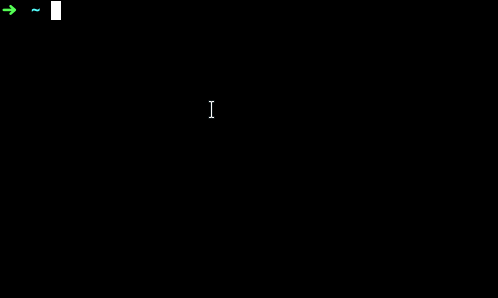### 函数的使用

``````# 传递一个字符串'hello world'作为参数给print函数
print('hello world!')

# 传递多个参数，默认以空格分开输出每个参数，其它类型的值自动转换成字符串类型
print('1', 2, '3')``````

``````>>> 2**3
8
>>> pow(2,3)
8
>>> ``````

``````>>> 1 + pow(2,10)
1025
>>> ``````

### 获取用户输入

``````print("下面计算a+b")

a = int(input("请输入a:"))
b = int(input("请输入b:"))

print("a + b = ", a+b)``````input函数接受一个字符串参数，并输出这个参数，等待用户的输入，按下回车后，输入的这一行字符串作为返回值返回出去。

``````>>> abs(-10) # 取绝对值
10
>>> round(1.5) # 四舍五入
2
>>> sum([1,2,3,4,5]) # 将一个列表或者类似的数据类型中的所有元素加起来
15
>>> ``````

round函数用于四舍五入，有时候我们需要把数值向下取整，我们可以利用`int()`转换成整数时截断小数来实现，也可以使用一个叫floor的函数实现，这个函数不能直接使用，它存在于一个模块中。

### 模块

``````>>> import math # 导入模块
>>> math.floor(1.9) # 使用math模块中的floor函数
1
>>> ``````

``````>>> from math import ceil
>>> ceil(12.1) # 向上取整
13
>>> ``````

``````# 把floor导入使用fl作为别名，把ceil导入并使用ce作为别名
from math import floor as fl,ceil as ce
print('fl(12.9) is', fl(12.9)) # 向下取整
print('ce(14.1) is', ce(14.1)) # 向上取整

# 导入math模块，使用别名m
import math as m
print('m.floor(12.9) is', m.floor(12.9))
print('m.ceil(14.1) is', m.ceil(14.1))

print('m.sqrt(4) is', m.sqrt(4)) # 计算4的平方根``````